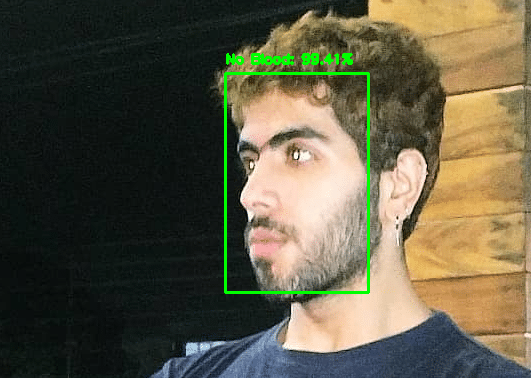# Blood Face Detector in Python (Part-1) — Machine Learning and Deep Learning Classification Project

Original article was published by MRINAL WALIA on Deep Learning on MediumFirst, we initialize the parameters for the model: learning rate, number of epochs to train for and batch size.

To train the model we have used the concept of Transfer Learning. We will fine-tune the MobileNet V2 architecture which is pre-trained on the ImageNet weights by leaving the head fully connected layer of the based model and then constructing our own head Fully Connected layer and place it on top of the base model.

During training, we freeze all the layers of the base model so that they don’t get updated during the first training process.

Then we compile the model using adam optimizer and binary cross-entropy loss function as it is a binary classification problem.

`# serialize the model to diskmodel.save('blood_noblood_classifier.model', save_format="h5")`

Then we save the model to disk using the model.save

## Step-4: Evaluate the performance of the Model

`# show a nicely formatted classification reportprint(classification_report(testY.argmax(axis=1), predIdxs, target_names=lb.classes_))# show a nicely formatted classification reportprint(confusion_matrix(testY.argmax(axis=1), predIdxs))# show a nicely formatted classification reportprint(accuracy_score(testY.argmax(axis=1), predIdxs))`

## Step-5: Plot of the training and validation accuracy and loss

`# plot the training loss and accuracyN = EPOCHSplt.style.use("ggplot")plt.figure()plt.plot(np.arange(0, N), H.history["loss"], label="train_loss")plt.plot(np.arange(0, N), H.history["val_loss"], label="val_loss")plt.plot(np.arange(0, N), H.history["acc"], label="train_acc")plt.plot(np.arange(0, N), H.history["val_acc"], label="val_acc")plt.title("Training Loss and Accuracy")plt.xlabel("Epoch #")plt.ylabel("Loss/Accuracy")plt.legend(loc="lower left")plt.savefig('model_plot.png')`

# Results:

As you can see, we are obtaining around 80% accuracy on our test set.

Given the noisy blood face images, we can think of improving the model accuracy by collecting more of the blood face dataset or by cleaning the dataset by some other approach.

Also, you are free to play around with the parameters and see how they affect the training accuracy

Given these results, we are hopeful that our model will generalize well to the validation dataset.

## Step-6: Make Predictions in Images

`# import the necessary packagesfrom tensorflow.keras.applications.mobilenet_v2 import preprocess_inputfrom tensorflow.keras.preprocessing.image import img_to_arrayfrom tensorflow.keras.models import load_modelimport numpy as npimport argparseimport matplotlib.pyplot as pltimport cv2import os# load our serialized face detector model from diskprototxtPath = os.path.sep.join(['Model', "deploy.prototxt"])weightsPath = os.path.sep.join(['Model', "res10_300x300_ssd_iter_140000.caffemodel"])net = cv2.dnn.readNet(prototxtPath, weightsPath)# load the blood face detector model from diskmodel = load_model('blood_noblood_classifier.model')# load the input image from disk, clone it, and grab the image spatial# dimensionsimage = cv2.imread(filename)orig = image.copy()(h, w) = image.shape[:2]# construct a blob from the imageblob = cv2.dnn.blobFromImage(image, 1.0, (300, 300), (104.0, 177.0, 123.0))# pass the blob through the network and obtain the face detectionsnet.setInput(blob)detections = net.forward()# loop over the detectionsfor i in range(0, detections.shape):    # extract the confidence (i.e., probability) associated with    # the detection    confidence = detections[0, 0, i, 2]    # filter out weak detections by ensuring the confidence is    # greater than the minimum confidence    if confidence > 0.6:        # compute the (x, y)-coordinates of the bounding box for        # the object        box = detections[0, 0, i, 3:7] * np.array([w, h, w, h])        (startX, startY, endX, endY) = box.astype("int")        # ensure the bounding boxes fall within the dimensions of        # the frame        (startX, startY) = (max(0, startX), max(0, startY))        (endX, endY) = (min(w - 1, endX), min(h - 1, endY))# extract the face ROI, convert it from BGR to RGB channel        # ordering, resize it to 224x224, and preprocess it        face = image[startY:endY, startX:endX]        face = cv2.cvtColor(face, cv2.COLOR_BGR2RGB)        face = cv2.resize(face, (224, 224))        face = img_to_array(face)        face = preprocess_input(face)        face = np.expand_dims(face, axis=0)        # pass the face through the model to determine if the face        # has a mask or not        (blood, noblood) = model.predict(face)# determine the class label and color we'll use to draw        # the bounding box and text        label = "Blood" if blood > noblood else "No Blood"        color = (0, 0, 255) if label == "Blood" else (0, 255, 0)        # include the probability in the label        label = "{}: {:.2f}%".format(label, max(blood, noblood) * 100)        # display the label and bounding box rectangle on the output        # frame        cv2.putText(image, label, (startX, startY - 10),            cv2.FONT_HERSHEY_SIMPLEX, 0.45, color, 2)        cv2.rectangle(image, (startX, startY), (endX, endY), color, 2)# show the output imagecv2.imshow("Output", image)cv2.waitKey(0)`